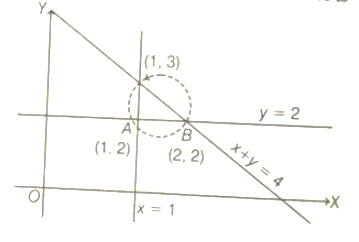Definition of a circle
Question

# A triangle is formed by the lines whose combined equation is given by (x+y-4)(x y-2 x-y+2)=0. The equation of its circumcircle is

Moderate
Solution

## therefore B C  is diameter, then equation of circumcircle is$⇒\left(\mathrm{x}-2\right)\left(\mathrm{x}-1\right)+\left(\mathrm{y}-2\right)\left(\mathrm{y}-3\right)=0$$⇒{\mathrm{x}}^{2}+{\mathrm{y}}^{2}-3\mathrm{x}-5\mathrm{y}+8=0$

Get Instant Solutions